Processing ......FreeComputerBooks.com Links to Free Computer, Mathematics, Technical Books all over the World

Bayesian Thinking (Bayesian Method, Bayesian Inference, etc.)
Related Book Categories:
 Probability and Stochastic Processes Machine Learning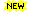Financial Mathematics and Engineering Applied Mathematics Algebra and Abstract Algebra Data Science•An Introduction to Bayesian Thinking (Merlise Clyde, et al.)

It provides an introduction to Bayesian Inference in decision making without requiring calculus. It may be used on its own as an open-access introduction to Bayesian inference using R Programming for anyone interested in learning about Bayesian statistics.

•Bayes Rules! An Introduction to Applied Bayesian Modeling

An engaging, sophisticated, and fun introduction to the field of Bayesian statistics, it brings the power of modern Bayesian thinking, modeling, and computing to a broad audience. Integrates R code, including RStan modeling tools, bayesrules package.

•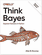O'Reilly® Think Bayes: Bayesian Statistics in Python

If you know how to program, you're ready to tackle Bayesian statistics. With this book, you'll learn how to solve statistical problems with Python code instead of mathematical formulas, using discrete probability distributions rather than continuous mathematics.

•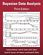Bayesian Data Analysis (Andrew Gelman, et al.)

This classic book is widely considered the leading text on Bayesian methods, lauded for its accessible, practical approach to analyzing data and solving research problems. It takes an applied approach to analysis using up-to-date Bayesian methods.

•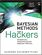Bayesian Methods for Hackers: Probabilistic Programming

This book illuminates Bayesian inference through probabilistic programming with the powerful PyMC language and the closely related Python tools NumPy, SciPy, Matplotlib, through practical examples and computation - no advanced mathematics required.

•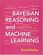Bayesian Reasoning and Machine Learning (David Barber)

This practical introduction is ideally suited to computer scientists without a background in calculus and linear algebra. You'll develop analytical and problem-solving skills that equip them for the real world. Numerous examples and exercises are provided.

•Uncertainty in Engineering: Bayesian and Monte Carlo Methods

This open access book provides an introduction to uncertainty quantiﬁcation in engineering. Starting with preliminaries on Bayesian statistics and Monte Carlo methods, it then focuses on reliability theory and simulation methods for complex systems.

•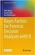Bayes Factors for Forensic Decision Analyses with R

This book provides a self-contained introduction to computational Bayesian statistics using R programming language. With its primary focus on Bayes factors supported by data sets, this book features an operational perspective, practical relevance, and applicability.

•Kalman and Bayesian Filters in Python (Roger R Labbe Jr.)

This book is an introductory text for Kalman and Bayesian filters. All code is written in Python, and the book itself is written using Juptyer Notebook so that you can run and modify the code in your browser. What better way to learn?

•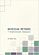Bayesian Methods for Statistical Analysis (Borek Puza)

Bayesian methods for statistical analysis is a book on statistical methods for analysing a wide variety of data. It contains many exercises, all with worked solutions, including complete computer code.

•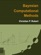Bayesian Computational Methods (Christian P. Robert)

This monograpg presents the most standard computational challenges met in Bayesian Statistics, focussing primarily on mixture estimation and on model choice issues, and then relate these problems with computational solutions.

•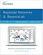Bayesian Networks and BayesiaLab (Stefan Conrady, et al.)

This practical introduction is geared towards scientists who wish to employ Bayesian Networks for applied research using the BayesiaLab software platform. It can serve as a self-study guide for learners and as a reference manual for advanced practitioners.

•Bayesian FreeBook (CRC)

This unique guide will help students develop the statistical confidence and skills to put the Bayesian formula into practice, from the basic concepts of statistical inference to complex applications of analyses.

•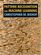Pattern Recognition and Machine Learning: Bayesian Viewpoint

This is the first textbook on pattern recognition to present the Bayesian viewpoint. The book presents approximate inference algorithms that permit fast approximate answers in situations where exact answers are not feasible.

•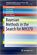Bayesian Methods in the Search for MH370 (Samuel Davey, et al.)

This book demonstrates how nonlinear/non-Gaussian Bayesian time series estimation methods were used to produce a probability distribution of potential MH370 flight paths, which was used to define the search zone in the southern Indian Ocean.

•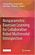Nonparametric Bayesian Learning for Collaborative Robot

This book introduces a fast, accurate, robot anomaly monitoring, diagnosis and recovery scheme for endowing robots with longer-term autonomy and a safer collaborative environment, emonstrates two robots that perform three manipulation tasks.

•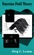Bayesian Field Theory (Jorg C. Lemm)

Long the province of mathematicians and statisticians, Bayesian methods are applied in this ground-breaking book to problems in cutting-edge physics, with practical examples of Bayesian analysis for the physicist working in such areas as neural networks, artificial intelligence, and inverse problems in quantum theory.

•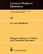Bayesian Spectrum Analysis and Parameter Estimation

This work is primarily on the application of probability theory to the parameter estimation problem. The people who will be interested in this material are physicists, economists, and engineers who have to deal with data on a daily basis; consequently.

•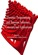Dynamic Programming and Bayesian Inference, Concepts/Apps

This is a book on the far-ranging algorithmic methododogy of Dynamic Programming. It presents a comprehensive and rigorous treatment of dynamic programming, and provides some applications of Bayesian optimization and dynamic programming.

Book Categories
 :All CategoriesTop BooksRecent BooksMiscellaneous BooksComputer LanguagesComputer ScienceData Science/DatabasesElectrical EngineeringJava and Java EE (J2EE)Linux and UnixMathematicsMicrosoft and .NETMobile ComputingNetworking and CommunicationsSoftware EngineeringSpecial TopicsWeb Programming
Other Categories